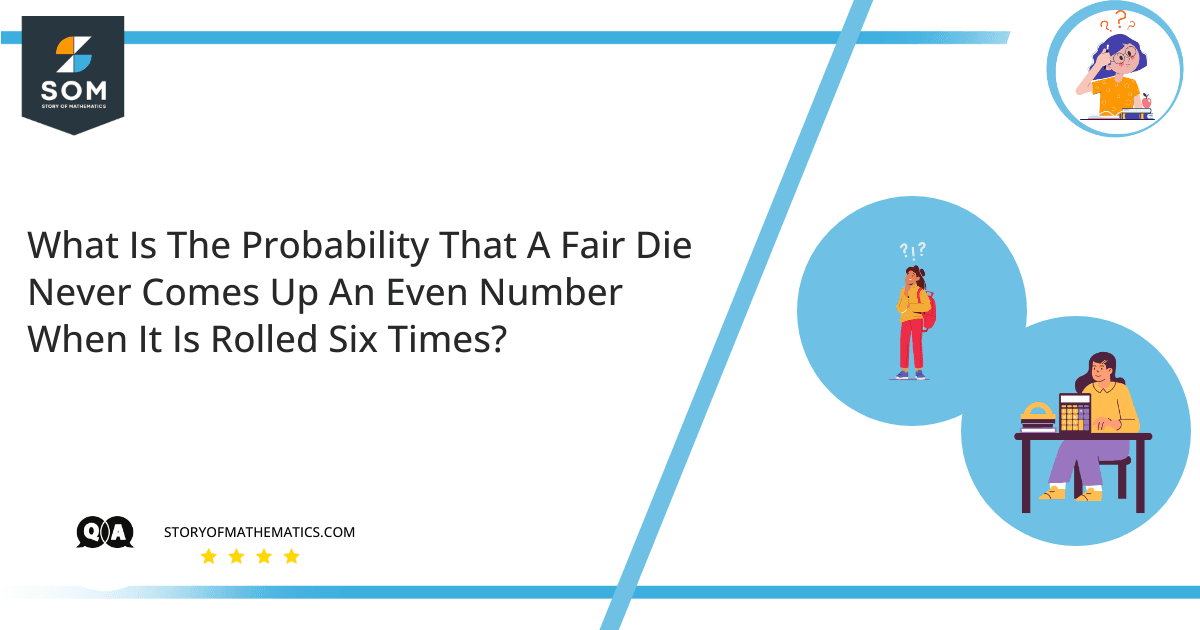# What is the probability that a fair die never comes up an even number when it is rolled six times?This problem aims to find the probability of the occurrence of a random event and its predictable outcomes. The concepts required for this problem are mainly related to probability and the product rule.

Let’s first look at a fair die, whose each face has the identical probability of coming faced up.

The product rule is stated as the probability of two autonomous events $(m,n)$ happening together can be estimated by multiplying the respective probabilities of each event arising independently $(m\times n)$.

So probability is a procedure to predict the happening of a random event, and its value is mostly between zero and one. It calculates the possibility of an event, events that are a bit tricky to anticipate an outcome.

Given as:

$\text{Probability of event to occur} = \dfrac{\text{Number of ways an event can occur}}{\text{Total number of outcomes of that event}}$

So as per the statement, a dice is rolled $6$ times and we are to find the probability that the outcome of these events is not an even number, or in other words, the outcome of these events is an odd number.

If we look at dice, we find a total of $6$ faces, of which only $3$ faces are odd, the rest are subsequently even numbers. Let’s create a sample space for a dice that is rolled only once:

$S_{\text{first role}}={1, 2, 3, 4, 5, 6}$

Out of which the odd numbers are:

$S_{odd}={1, 3, 5 }$

So the probability of getting an odd number with a single role is:

$P_{1 role}(O)=\dfrac{\text{Odd faces}}{\text{Total faces}}$

$P_{1 role}(O)=\dfrac{3}{6}$

$P_{1 role}(O)=\dfrac{1}{2}$

So the probability that the number would be odd after the first role is $0.5$.

Similarly, in every role there are a total of $6$ outcomes:

$S_{2^{nd} … 6^{th}} = {1, 2, 3, 4, 5, 6}$

Here we are going to use the property of the product rule to calculate the total number of outcomes after six roles:

$\text{Total outcomes}=6\times 6\times 6\times 6\times 6\times 6$

$\text{Total outcomes}=6^6 = 46656$

Since there are only $3$ odd numbers in a die, the total number of outcomes becomes:

$\text{Odd outcomes} = 3\times 3\times 3\times 3\times 3\times 3$

$\text{Odd outcomes} = 3^6 = 729$

So $729$ of the $46656$ outcomes results in an odd number.

Now the probability becomes:

$P_{6\space roles}(O)=\dfrac{729}{46656}$

$P_{6\space roles}(O)=0.0156$

## Numerical Result

The probability that the outcome of a fair die rolled six times would not be an even number is $0.0156$.

## Example

A dice is rolled six times, find the probability of getting the number six.

Let’s assume $P$ is the probability of getting a $6$:

$P=\dfrac{1}{6}$

Similarly, the probability of getting any number other than $6$ is:

$P’= 1-P=\dfrac{5}{6}$

Now we are going to use the property of the product rule to calculate the total number of outcomes after six roles:

$\text{P(Not getting a 6 for n times)} = \text{P’ to the n_{th} power}$

So it becomes:

$(\dfrac{5}{6})^6 = \dfrac{15,625}{46,656} \approx 0.334$

Hence, the probability of getting a six at least once is $1-0.334=0.666$.